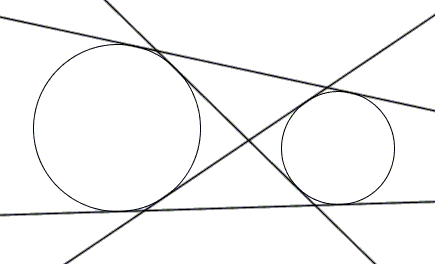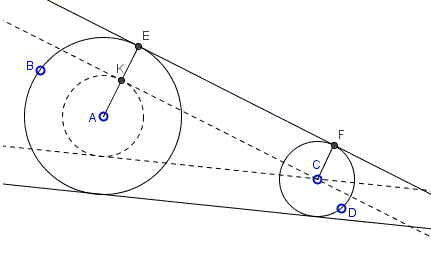# How to Construct Common Tangents to Two Circles

Given circles $A(B)$ and $C(D)$, construct the lines tangent to the two circles.Solution

Below is an applet that is supposed to be suggestive of the construction:Given circles $A(B)$ and $C(D)$, construct the lines tangent to the two circles.As is often the case in mathematics, the sought construction of common tangents two to circles is reduced to a simpler construction of tangents from a point to a circle.

To see how it works, let's introduce notation $R_c$for the radius of circle $c$. Assume (without loss of generality) that $R_{(A)}\ge R_{(B)}.$ Form an auxiliary circle $(A)'$ with center $A$ and radius $R_{(A)}\ge R_{(B)}.$Tangents to a circle are perpendicular to the radii at the points of contact. Thus, in the diagram, if $CK$ is tangent to $(A)'$ then $AK\perp CK.$ If $E$ is the intersection of $AK$ with $A(B)$ and $AK||CF$, with $F$ on $C(D)$, then, first of all $AE$ and $CF$ are radii of $A(B)$ and $C(D),$ respectively. Also, by the construction, $KE=CF,$ $KE||CF,$, and the angle at $K$ is right, making $ACFE$ a rectangle. It follows that the radii $AE$ and $CF$ are perpendicular to $EF$, implying tat the latter is tangent to both circles.

To construct the inner tangents, reduce first $C(D)$ to a point and simultaneously expand $A(B)$ by $R_{C(D)}.$ The argument is about the same as before.Several cases are possible. If the circles coincide, the number of common tangents is infinite - one per every point on the circle. If one of the circles lies entirely within the other, they have no common tangents. If they touch internally, their single common tangent can be said to play a double role. If the circles intersect, they have two outer tangents. If they touch externally, there are two outer tangents and one tangent at their common point. Otherwise, there are two outer and two inner tangents.

When two circles are tangent externally, their common tangents are found to be in an interesting relationship.### Various Geometric Constructions

• How to Construct a Radical Axis
• Constructions Related To An Inaccessible Point
• Inscribing a regular pentagon in a circle - and proving it
• The Many Ways to Construct a Triangle and additional triangle facts
• Easy Construction of Bicentric Quadrilateral
• Easy Construction of Bicentric Quadrilateral II
• Star Construction of Shapes of Constant Width
• Four Construction Problems
• Geometric Construction with the Compass Alone
• Construction of n-gon from the midpoints of its sides
• Short Construction of the Geometric Mean
• Construction of a Polygon from Rotations and their Centers
• Squares Inscribed In a Triangle I
• Construction of a Cyclic Quadrilateral
• Circle of Apollonius
• Six Circles with Concurrent Pairwise Radical Axes
• Trisect Segment: 2 Circles, 4 Lines
• Tangent to Circle in Three Steps
• Regular Pentagon Construction by K. Knop
•